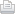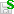https://doi.org/10.5194/npg-2018-35
https://doi.org/10.5194/npg-2018-35
Status: this preprint was under review for the journal NPG but the revision was not accepted.

# Asymptotes of the nonlinear transfer and wave spectrum in the frame of the kinetic equation solution

Vladislav G. Polnikov, Fangli Qiao, and Yong Teng

Abstract. The kinetic equation for a gravity wave spectrum is solved numerically to study the high frequencies asymptotes for the one-dimensional nonlinear energy transfer and the variability of spectrum parameters that accompany the long-term evolution of nonlinear waves. The cases of initial two-dimensional spectra S(ω,θ) of modified JONSWAP type with the frequency decay-law S(ω) ~ ω−n (for n = 6, 5, 4 and 3.5) and various initial functions of the angular distribution are considered. It is shown that at the first step of the kinetic equation solution, the nonlinear energy transfer asymptote has the power-like decay-law, Nl(ω) ~ ω−p, with values p ≤ n − 1, valid in cases when n ≥ 5, and the difference, n-p, changes significantly when n approaches 4. On time scales of evolution greater than several thousands of initial wave periods, in every case, a self-similar spectrum Ssf(ω,θ) is established with the frequency decay-law of form S(ω) ~ ω−4. Herein, the asymptote of nonlinear energy transfer becomes negative in value and decreases according to the same law (i.e., Nl(ω) ~ −ω−4). The peak frequency of the spectrum, ωp(t), migrates in time t to the low-frequency region such that the angular and frequency characteristics of the two-dimensional spectrum Ssf(ω,θ) remain constant. However, these characteristics depend on the degree of angular anisotropy of the initial spectrum. The solutions obtained are interpreted, and their connection with the analytical solutions of the kinetic equation by Zakharov and co-authors for gravity waves in water is discussed.

Share

Status: closed
Status: closed
AC: Author comment | RC: Referee comment | SC: Short comment | EC: Editor comment- Printer-friendly version- Supplement

Status: closed
Status: closed
AC: Author comment | RC: Referee comment | SC: Short comment | EC: Editor comment- Printer-friendly version- Supplement

## Viewed

Total article views: 2,170 (including HTML, PDF, and XML)
HTML PDF XML Total BibTeX EndNote
1,756 337 77 2,170 74 73
• HTML: 1,756
• PDF: 337
• XML: 77
• Total: 2,170
• BibTeX: 74
• EndNote: 73